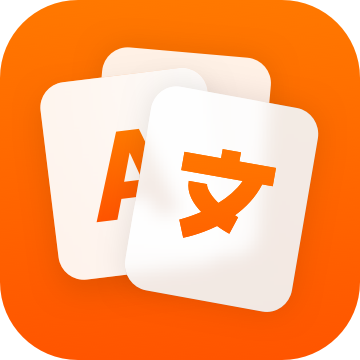By using this site, you agree that we're using cookies to save preferences and for telemetry data such as Google Analytics.

Indicative

Present Simple
Perfect Simple
Past Simple
Pluperfect Simple
Future Simple
Future perfect Simple
Present Continuous
Perfect Continuous
Past Continuous
Pluperfect Continuous
Future Continuous
Future perfect Continuous
 I bound you bound he/she/it bounds we bound they bound
 I have bounded you have bounded he/she/it has bounded we have bounded they have bounded
 I bounded you bounded he/she/it bounded we bounded they bounded
 I will bound you will bound he/she/it will bound we will bound they will bound
 I will have bounded you will have bounded he/she/it will have bounded we will have bounded they will have bounded
 I am bounding you are bounding he/she/it is bounding we are bounding they are bounding
 I have been bounding you have been bounding he/she/it has been bounding we have been bounding they have been bounding
 I was bounding you were bounding he/she/it was bounding we were bounding they were bounding
 I had been bounding you had been bounding he/she/it had been bounding we had been bounding they had been bounding
 I will be bounding you will be bounding he/she/it will be bounding we will be bounding they will be bounding
 I will have been bounding you will have been bounding he/she/it will have been bounding we will have been bounding they will have been bounding

Subjunctive

Present Simple
Perfect Simple
Imperfect Simple
Pluperfect Simple
 I bound you bound he/she/it bound we bound they bound
 I have bounded you have bounded he/she/it have bounded we have bounded they have bounded
 I bounded you bounded he/she/it bounded we bounded they bounded

Conditional

Present Simple
Perfect Simple
Present Continuous
Perfect Continuous
 I would bound you would bound he/she/it would bound we would bound they would bound
 I would have bounded you would have bounded he/she/it would have bounded we would have bounded they would have bounded
 I would be bounding you would be bounding he/she/it would be bounding we would be bounding they would be bounding
 I would have been bounding you would have been bounding he/she/it would have been bounding we would have been bounding they would have been bounding

Imperative

 you bound we Let's bound

← Conjugate another English verbLearn languages with Reji.

One-time purchase for a reasonable price.
No subscriptions, no hidden costs.